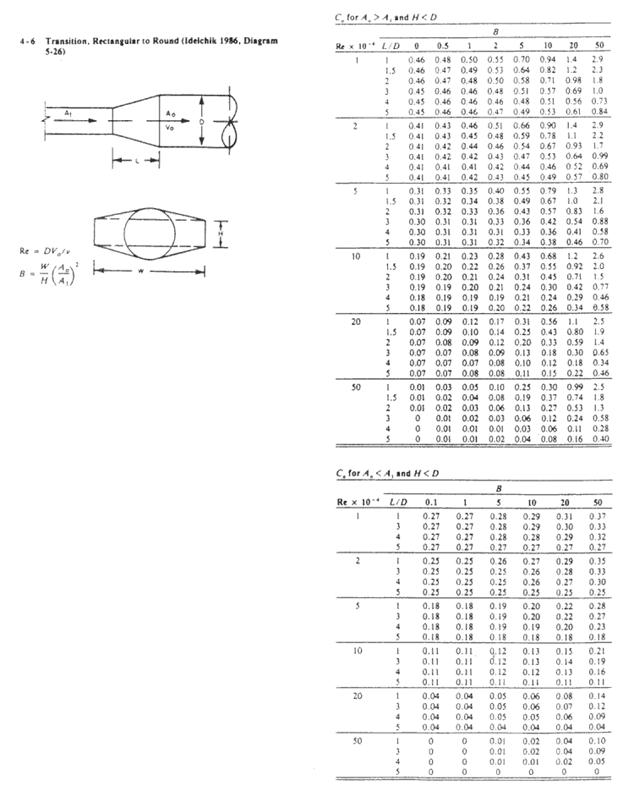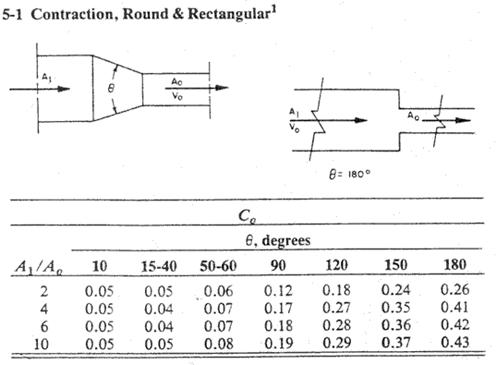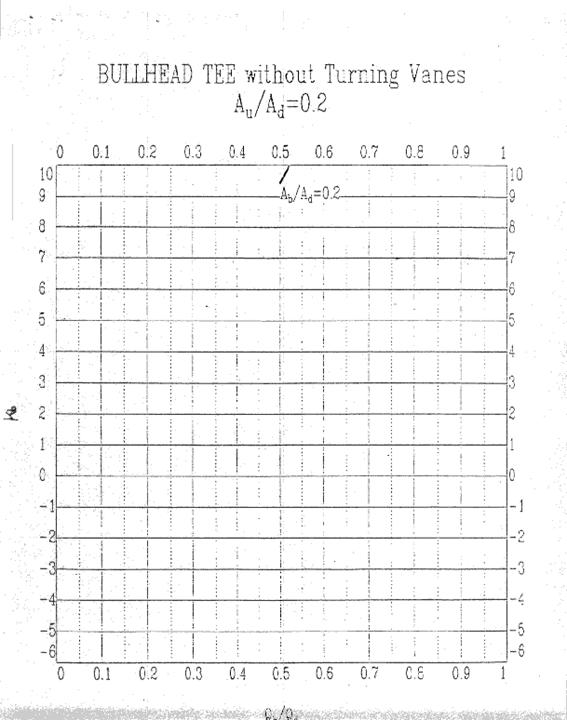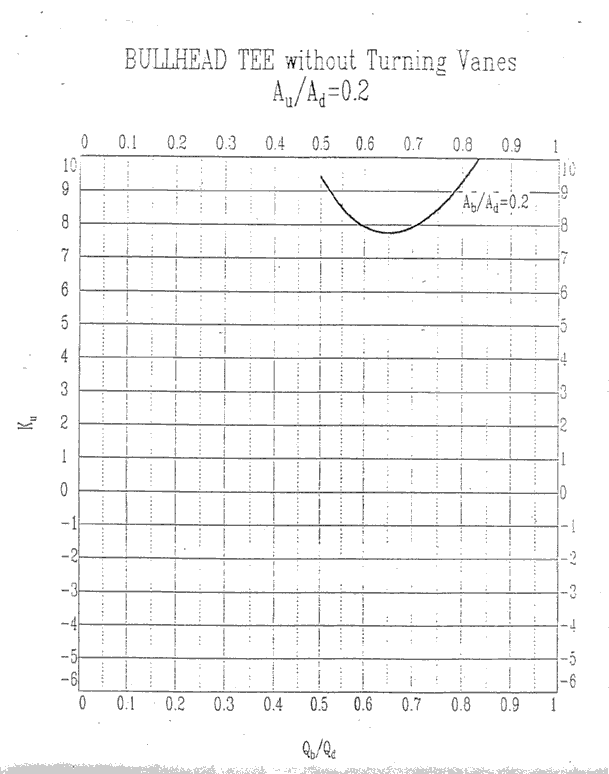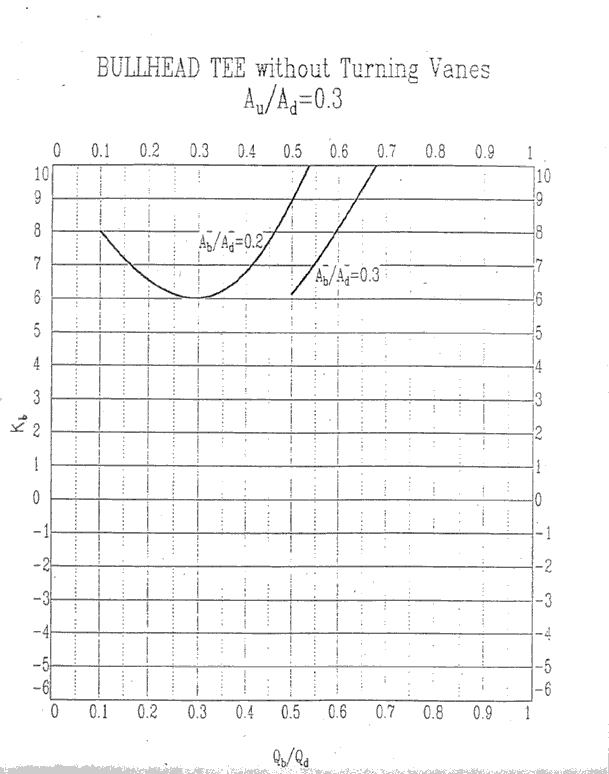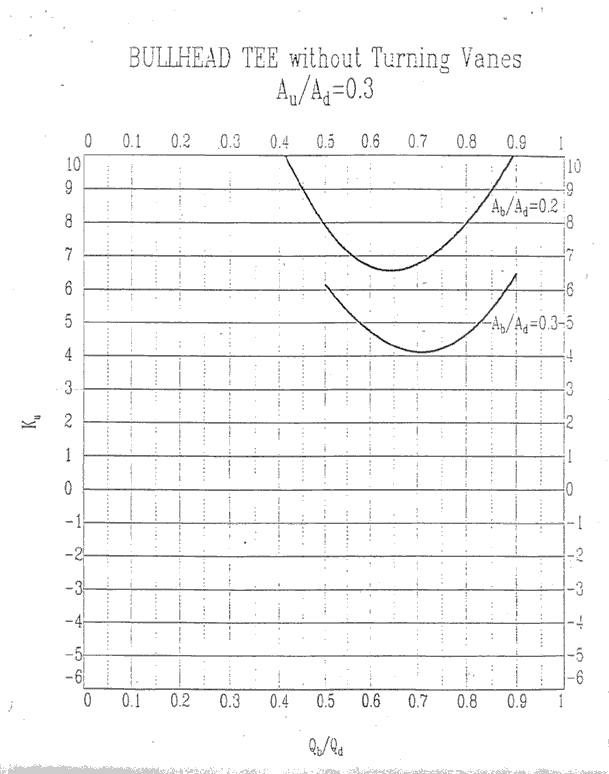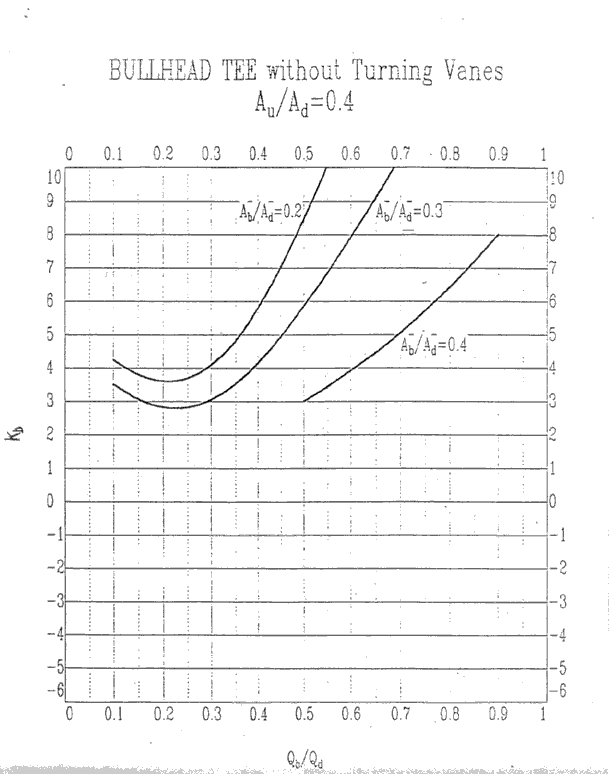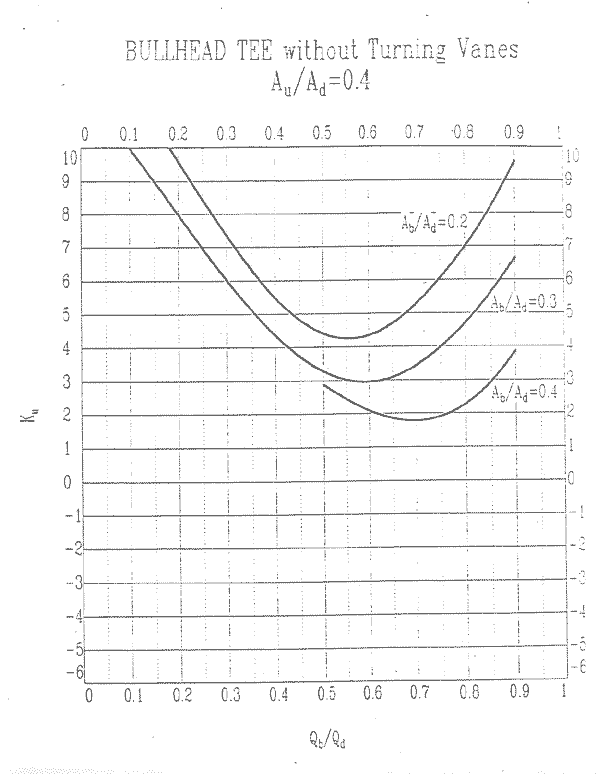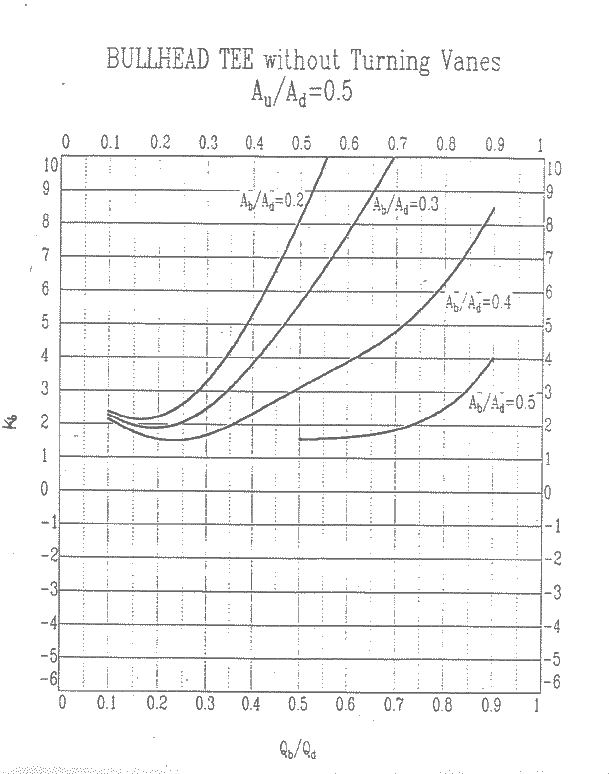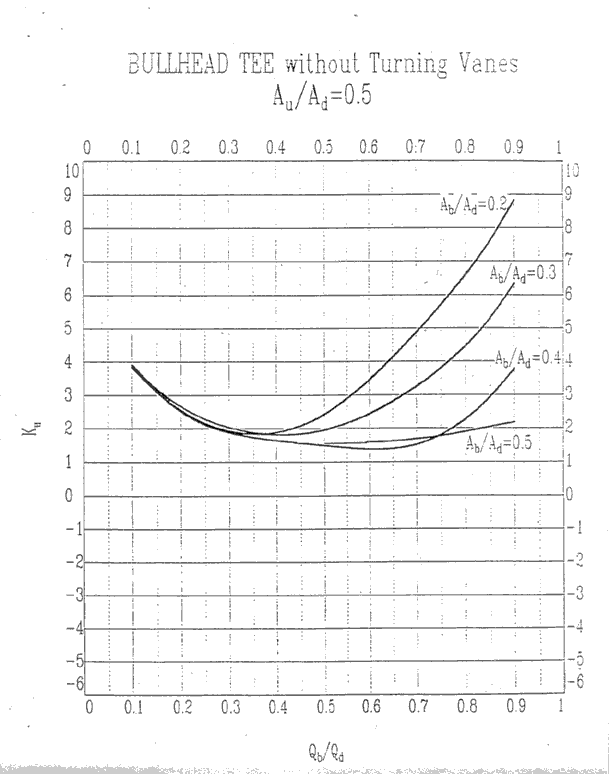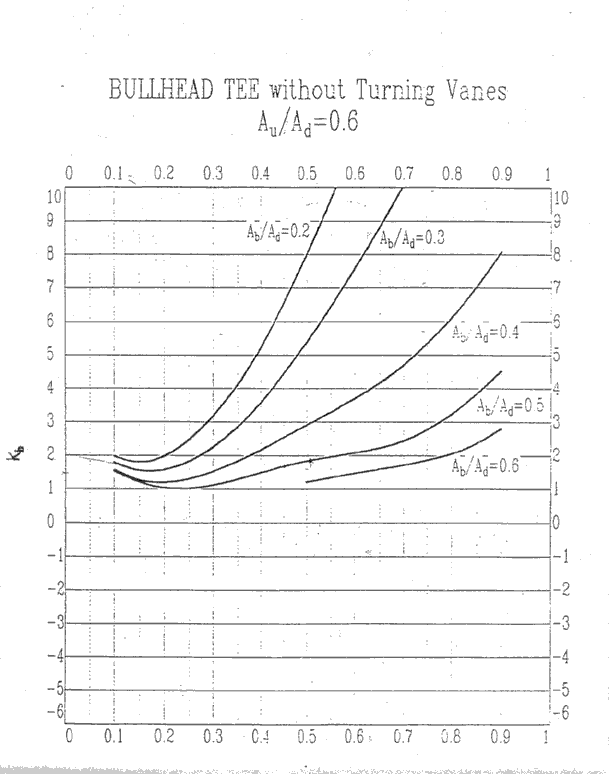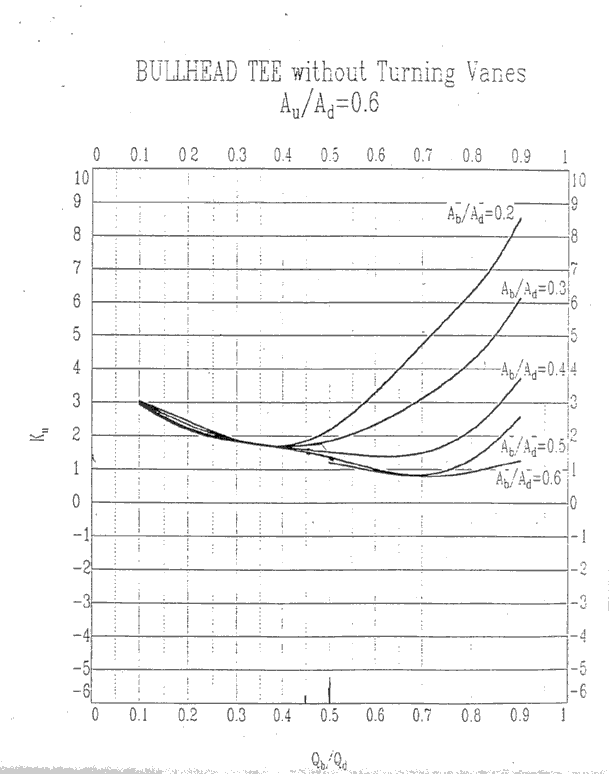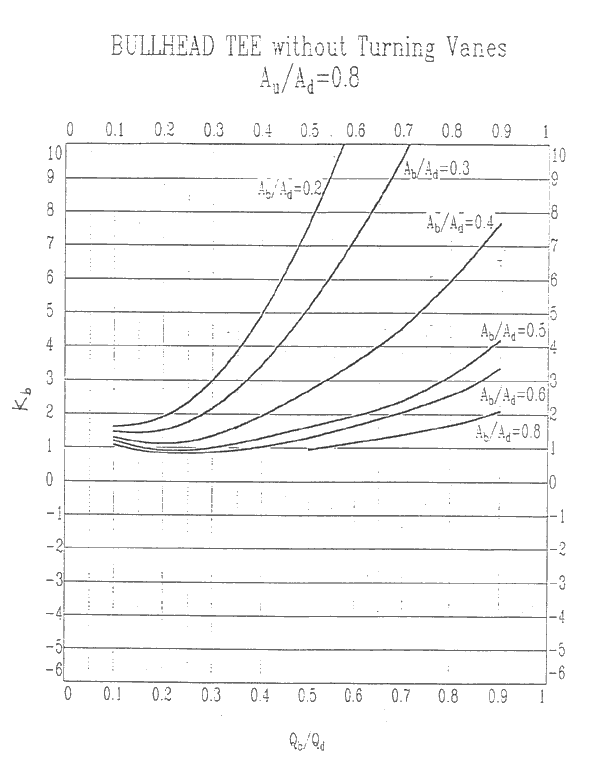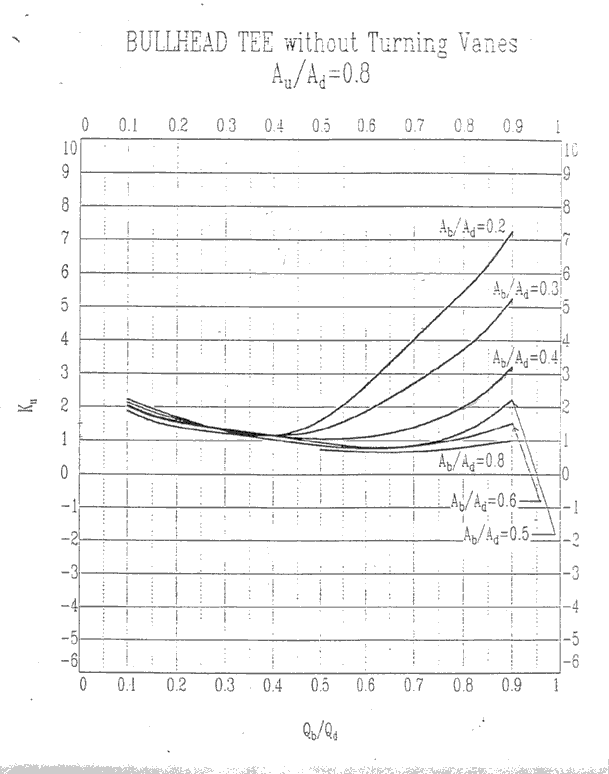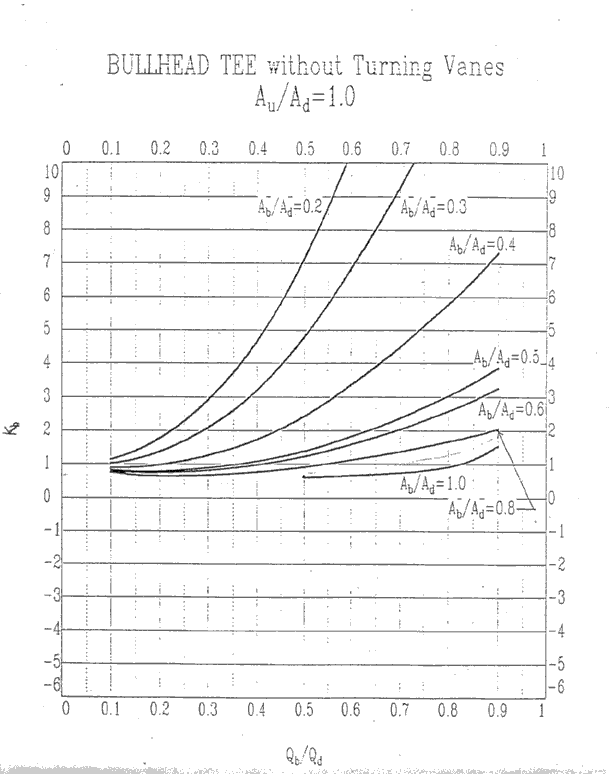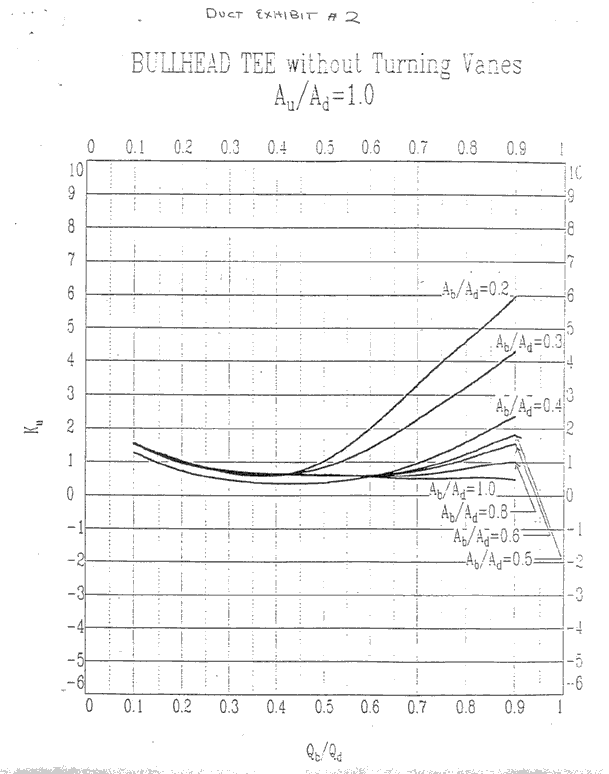Designing Air Flow Systems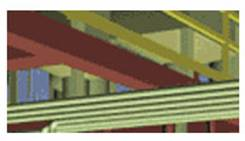A theoretical and practical guide to the basics of designing air flow systems. 1.          1.1.        1.2.        1.3.        2.          2.1.        2.2.        2.3.        3.          3.1.        3.2.        3.3.        4.          4.1.        4.2.        5.          5.1.        5.2.        6.          7.          8.          9.          10.       11.     Appendix 3 – Bullhead Tee Curves

1.  Air Flow

Flow of air or any other fluid is caused by a pressure differential between two points.  Flow will originate from an area of high energy, or pressure, and proceed to area(s) of lower energy or pressure.Duct air moves according to three fundamental laws of physics: conservation of mass, conservation of energy, and conservation of momentum.

Conservation of mass simply states that an air mass is neither created nor destroyed. From this principle it follows that the amount of air mass coming into a junction in a ductwork system is equal to the amount of air mass leaving the junction, or the sum of air masses at each junction is equal to zero. In most cases the air in a duct is assumed to be incompressible, an assumption that overlooks the change of air density that occurs as a result of pressure loss and flow in the ductwork. In ductwork, the law of conservation of mass means a duct size can be recalculated for a new air velocity using the simple equation:

V2 = (V1 * A1)/A2
Where V is velocity and A is Area

The law of energy conservation states that energy cannot disappear; it is only converted from one form to another. This is the basis of one of the main expression of aerodynamics, the Bernoulli equation. Bernoulli's equation in its simple form shows that, for an elemental flow stream, the difference in total pressures between any two points in a duct is equal to the pressure loss between these points, or:

(Pressure loss)1-2 = (Total pressure)1 - (Total pressure)2

Conservation of momentum is based on Newton's law that a body will maintain its state of rest or uniform motion unless compelled by another force to change that state. This law is useful to explain flow behavior in a duct system's fitting.

Laminar Flow

Flow parallel to a boundary layer.  In HVAC system the plenum is a duct.

Turbulent Flow
Flow which is perpendicular and near the center of the duct and parallel near the outer edges of the duct.

Most HVAC applications fall in the transition range between laminar and turbulent flow.

Pressure loss is the loss of total pressure in a duct or fitting. There are three important observations that describe the benefits of using total pressure for duct calculation and testing rather than using only static pressure.

·        Only total pressure in ductwork always drops in the direction of flow. Static or dynamic pressures alone do not follow this rule.

·        The measurement of the energy level in an air stream is uniquely represented by total pressure only. The pressure losses in a duct are represented by the combined potential and kinetic energy transformation, i.e., the loss of total pressure.

·        The fan energy increases both static and dynamic pressure. Fan ratings based only on static pressure are partial, but commonly used.

Pressure loss in ductwork has three components, frictional losses along duct walls and dynamic losses in fittings and component losses in duct-mounted equipment.

Component Pressure

Due to physical items with known pressure drops, such as hoods, filters, louvers or dampers.

Dynamic Pressure

Dynamic losses are the result of changes in direction and velocity of air flow. Dynamic losses occur whenever an air stream makes turns, diverges, converges, narrows, widens, enters, exits, or passes dampers, gates, orifices, coils, filters, or sound attenuators. Velocity profiles are reorganized at these places by the development of vortexes that cause the transformation of mechanical energy into heat. The disturbance of the velocity profile starts at some distance before the air reaches a fitting. The straightening of a flow stream ends some distance after the air passes the fitting. This distance is usually assumed to be no shorter then six duct diameters for a straight duct. Dynamic losses are proportional to dynamic pressure and can be calculated using the equation:

Dynamic loss = (Local loss coefficient) * (Dynamic pressure)

where the Local loss coefficient, known as a C-coefficient, represents flow disturbances for particular fittings or for duct-mounted equipment as a function of their type and ratio of dimensions. Coefficients can be found in the ASHRAE Fittings diagrams.

A local loss coefficient can be related to different velocities; it is important to know which part of the velocity profile is relevant. The relevant part of the velocity profile is usually the highest velocity in a narrow part of a fitting cross section or a straight/branch section in a junction.

Frictional Pressure
Frictional losses in duct sections are result from air viscosity and momentum exchange among particles moving with different velocities.  These losses also contribute negligible losses or gains in air systems unless there are extremely long duct runs or there are significant sections using flex duct.

The easiest way of defining frictional loss per unit length is by using the Friction Chart (ASHRAE, 1997); however, this chart (shown below) should be used for elevations no higher of 500 m (1,600 ft), air temperature between 5°C and 40°C (40°F and 100°F), and ducts with smooth surfaces.  The Darcy-Weisbach Equation should be used for “non-standard” duct type such as flex duct.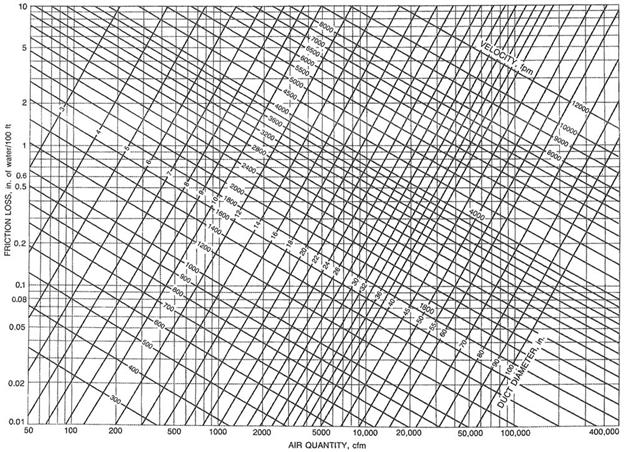Friction Chart   (ASHRAE HANDBOOK, 1997)

It is convenient to calculate pressures in ducts using as a base an atmospheric pressure of zero. Mostly positive pressures occur in supply ducts and negative pressures occur in exhaust/return ducts; however, there are cases when negative pressures occur in a supply duct as a result of fitting effects.

Airflow through a duct system creates three types of pressures: static, dynamic (velocity), and total. Each of these pressures can be measured. Air conveyed by a duct system imposes both static and dynamic (velocity) pressures on the duct's structure. The static pressure is responsible for much of the force on the duct walls. However, dynamic (velocity) pressure introduces a rapidly pulsating load.

Static pressure
Static pressure is the measure of the potential energy of a unit of air in the particular cross section of a duct. Air pressure on the duct wall is considered static. Imagine a fan blowing into a completely closed duct; it will create only static pressure because there is no air flow through the duct.  A balloon blown up with air is a similar case in which there is only static pressure.

Dynamic (velocity) pressure
Dynamic pressure is the kinetic energy of a unit of air flow in an air stream. Dynamic pressure is a function of both air velocity and density:

Dynamic pressure = (Density) * (Velocity)2 / 2

The static and dynamic pressures are mutually convertible; the magnitude of each is dependent on the local duct cross section, which determines the flow velocity.

Total Pressure

Consists of the pressure the air exerts in the direction of flow (Velocity Pressure) plus the pressure air exerts perpendicular to the plenum or container through which the air moves.  In other words:

PT = PV + PS

PT = Total Pressure

PV = Velocity Pressure

PS =  Static Pressure

This general rule is used to derive what is called the Fan Total Pressure.  See the section entitled Fan Performance Specifications for a definition of Fan Total Pressure and Fan Static Pressure.

For kitchen ventilation applications an air system consists of hood(s), duct work, and fan(s).  The relationship between the air flow rate (CFM) and the pressure of an air system is expressed as an increasing exponential function.  The graph below shows an example of a system curve.  This curve shows the relationship between the air flow rate and the pressure of an air system.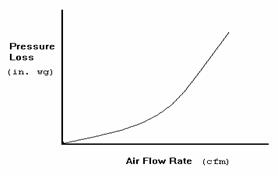Complex systems with branches and junctions, duct size changes, and other variations can be broken into sections or sub-systems.  Each section or sub-system has its own system curve.  See the diagram below for an illustration of this concept.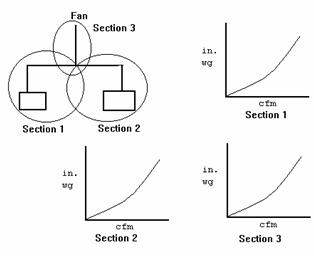Use the Fan Laws along a system curve.  If you know one (CFM, S.P.) point of a system you could use Fan Law 2 to determine the static pressure for other flow rates.  They apply to a fixed air system.  Once any element of the system changes, duct size, hood length, riser size, etc.. the system curve changes.

CFM x        RPM x                                                              Fan Law 1

-------   =     -------

CFM known   RPM known

SP x        CFM2 x        RPM2x                                                            Fan Law 2

------  =   -------   =     -------

SP known   CFM2known  RPM2known

BHPx       CFM3x        RPM3x                                                            Fan Law 3

------  =   -------   =      -------

BHPknown CFM3known  RPM3known

Other calculations can be utilized to maneuver around a fan performance curve.  For example, to calculate BHP from motor amp draw, use the following formula:

1 phase motors                                 3 phase motors

BHP = V * I * E * PF                       BHP = V * I * E * PF * 1.73

746                                                      746

where:
BHP = Brake Horsepower
V = Line Voltage
I = Line Current
E = Motor Efficiency (Usually about .85 to .9)
PF = Motor Power Factor (Usually about .9)

Once the BHP is known, the RPM of the fan can be measured.  The motor BHP and fan RPM can then be matched on the fan performance curve to approximate airflow.

2.2.  Air Density

The most common influences on air density are the effects of temperature other than 70 °F and barometric pressures other than 29.92” caused by elevations above sea level.

Ratings found in fan performance tables and curves are based on standard air.  Standard air is defined as clean, dry air with a density of 0.075 pounds per cubic foot, with the barometric pressure at sea level of 29.92 inches of mercury and a temperature of 70 °F.  Selecting a fan to operate at conditions other then standard air requires adjustment to both static pressure and brake horsepower.  The volume of air will not be affected in a given system because a fan will move the same amount of air regardless of the air density. In other words, if a fan will move 3,000 cfm at 70 °F it will also move 3,000 CFM at 250 °F.  Since 250 °F air weighs only 34% of 70°F air, the fan will require less BHP but it will also create less pressure than specified.

When a fan is specified for a given CFM and static pressure at conditions other than standard, the correction factors (shown in table below) must be applied in order to select the proper size fan, fan speed and BHP to meet the new condition.

The best way to understand how the correction factors are used is to work out several examples.  Let’s look at an example using a specification for a fan to operate at 600°F at sea level.  This example will clearly show that the fan must be selected to handle a much greater static pressure than specified.

Example #1:  A 20” centrifugal fan is required to deliver 5,000 cfm at 3.0 inches static pressure. Elevation is 0 (sea level).  Temperature is 600°F.  At standard conditions, the fan will require 6.76 bhp

1.            Using the chart below, the correction factor is 2.00.

2.            Multiply the specified operating static pressure by the correction factor to determine the standard air density equivalent static pressure.  (Corrected static pressure = 3.0 x 2.00 = 6”.  The fan must be selected for 6 inches of static pressure.)

3.            Based upon the performance table for a 20 fan at 5,000 cfm at 6 inches wg, 2,018 rpm is needed to produce the required performance.

4.            What is the operating bhp at 600 °F?

Since the horsepower shown in the performance chart refers to standard air density, this should be corrected to reflect actual bhp at the lighter operating air.

Operating bhp = standard bhp
¸ 2.00 or 6.76 ¸ 2.00 = 3.38 bhp.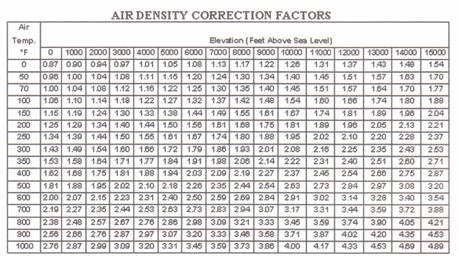2.3.  System Constant

Every air system or sub-system has a system constant.  This constant can be calculated as long as you know one (CFM, Static Pressure) point.   You use a variation of the fan laws to calculate the system constant.  To calculate the system constant:

K system = S.P./(CFM)2

Once you have the system constant you can calculate the static pressure for any flow rate.

S.P. = (CFM)2 * K system

Pressure losses are more easily determined by breaking an air system into sections.  Sections can be in series or in parallel.

3.1.  Sections in Series

For sections or components in series simply sum up all the sections. A single duct that has the same shape, cross section, and mass flow is called a duct section or just a section.

Following is the recommended procedure for calculating total pressure loss in a single duct section:

·        Gather input data: air flow, duct shape, duct size, roughness, altitude, air temperature, and fittings;

·        Calculate air velocity as a function of air flow and cross section;

·        Calculate local C-coefficients for each fitting used; and

·        Calculate pressure loss using the friction chart

The following is a simple example of how duct pressure accumulates and is totaled in a section.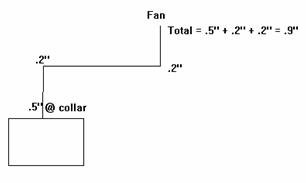3.2.  Sections in Parallel

When designing sections that are parallel it is important to remember that the branches of a junction all have the same total pressure.  This is a fact.  It is governed by a principle which states that areas of high energy move to areas of lower energy.  We will see how this applies to air systems in parallel.

To illustrate these concepts we will reference the diagram below.  In this example we calculate the pressure losses for Section 1 to be -0.75” at the junction.  We calculate the pressure losses for Section 2 to be -0.6” at the junction. (NOTE: For simplicity’s sake we do not consider the pressure loss incurred by the junction.)  These would be the actual pressure losses of the system were they operating independently; however, they do not.  They interact at the junction.  This means that whenever air flow encounters a junction it will take the path of least resistance and the total pressure losses of each branch of the junction will be the same.

For sections that run parallel, always use the section with the higher pressure loss/gain to determine pressure losses/gains through a system.  Adjust the branch with the lower pressure loss/gain by increasing the flow rate or decreasing the duct size to increase the pressure loss to that of the higher branch.

If the flow rate or the duct size is not changed the air flow through each branch will adjust itself so that each branch has the same total pressure loss/gain.  In other words, more air flows through the branch with the lower pressure loss/gain or energy state.

In the example below, the actual pressure loss would be somewhere between -0.75” and -0.6”.  Section 1 would pull less than 2000 CFM and Section 2 would pull more than 1800 CFM.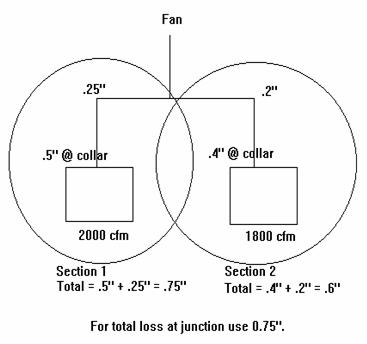3.3.  System Effect

System Effect occurs in an air system when two or more elements such as fittings, a hood and a fitting, or a fan and a fitting occur within close proximity to one another.  The effect is to increase the energy or pressure in a system as air flows through the elements.  To calculate the pressure loss incurred by such a configuration, consider two elements at a time.  For example, if two elbows occur 4 feet from one another this configuration will have a pressure loss associated with it.

Calculate the pressure loss/gain associated with each fitting as if it occurs alone.  Sum these and multiply them by a system effect coefficient (K).  The system effect coefficient can be obtained from the ASHRAE Fitting Diagrams for only a limited number of configurations of elements.

Configurations not listed must use estimates or best guesses.  In many cases, you can use a listed configuration as a guide.

One configuration not listed is an elbow within close proximity to the collar of a hood.  As a rule of thumb, the chart below can offer some guidance for determining the system effect for this situation.  Remember the coefficients in the chart are only an estimate.

 Distance between Riser and Elbow System Effect Coefficient (K) 2 feet 1.75 3 feet 1.5 4 feet 1.3 5 feet 1.2

The diagrams below show system effect factors for straight through elements and turning elements.  For rectangular ductwork, D = (2HW)/(H+W).  The following formula should be used to calculate the pressure caused by system effect:

Pressure Loss = K * (Element A Resistance + Element B Resistance)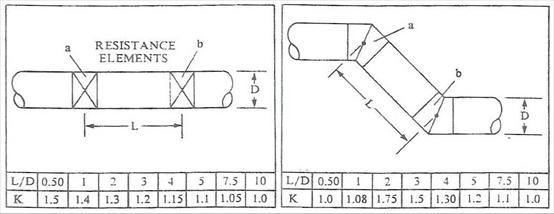Straight Through Flow                                       Turning Elements

The following diagrams show proper and improper methods of constructing ductwork: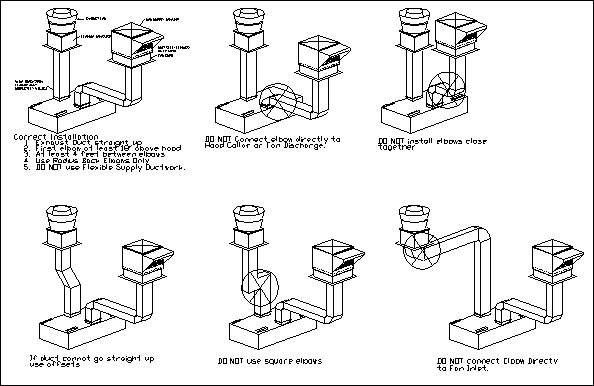A fan performance spec is given as a Fan Total Pressure or a Fan Static Pressure which can handle a certain flow rate.  Most manufacturers' performance charts are based on Fan Static Pressure.

Fan total Pressure is the pressure differential between the inlet and the outlet of the fan.  It can be expressed in these terms:

P t fan = P t loss + P v system outlet + (P s system outlet + P s system entry + P v system entry)

P t fan = Fan Total Pressure

P t loss = Dynamic, Component, and Frictional Pressure through the air system.

P s  system outlet = Static Pressure at System Outlet

P s  system entry = Static Pressure at System Entry

P v  system entry = Velocity Pressure at System Entry

P v  system  outlet = Velocity Pressure at System Outlet

For most HVAC applications:  (P s outlet + P s entry + P v entry)  = 0

Therefore:  P t fan = P t loss + P v system  outlet

The Fan Static Pressure is expressed as the Fan Total Pressure minus the velocity pressure at the fan discharge, or:

P s fan = P t loss + P v  system  outlet - P v discharge

Where P v discharge = Velocity Pressure at the Fan Discharge.

For Exhaust Systems with resistance only on the inlet side, the fan static pressure is:

P s fan = P t loss

For exhaust system:   P v system  outlet = P v discharge

For Supply Systems with resistance on the outlet side, the fan static pressure is:

P s fan = P t loss - P v discharge

P v system outlet can be assumed to be 0.

The diagram below illustrates the difference between exhaust and supply systems.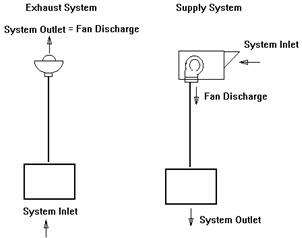5.1.  Methodology

Break the system into sections.

A new section occurs at:

1) Changes in duct size.

2)  Change in air volume

Calculate losses for each section.

Begin at the section farthest from the fan and work towards the fan.  For each section:

1.            Write down or calculate all known variables.

Air Flow Rate. (Q)

Duct Cross-Sectional Area of the section. (A)

Center-Line Length of the section.  (L)

Air Velocity through the section. (V=Q/A)

Velocity Pressure.  (Pv = (V/4005)2)

2.            Write down or calculate all pressure losses in the section.

a)  List the Component Losses/Gains.

Incurred by hoods, ESPs, filters, dampers, etc..

b)  Calculate the Dynamic Losses/Gains.

Occur through elbows, transitions, tees, or any other type of fitting.

Use the ASHRAE Fitting Diagrams to find Dynamic Loss Coefficients for fittings.

Be sure to factor in System Effect!

c)  Calculate Frictional Losses/Gains.

Use the ASHRAE Friction Chart for “standard” galvanized ductwork.

Use the Darcy-Weisbach Equation for “non-standard” duct such as flex duct.

3.                Sum up the Component, Dynamic, and Frictional Pressure for the section.

4.                Sum up the pressure losses for all of the sections.

Standard Air Density, .075 lb/cu ft, is used for most HVAC applications.

Frictional losses based on galvanized metal duct with 40 joints per 100 ft.

Correction for "Non-Standard" Duct Material

If material other than galvanized metal is used in parts of the system, you will have to adjust for the difference in the material's roughness factor.  This means the Friction Chart typically used to determine frictional losses cannot be used and you must use a variation of the Darcy-Weisbach Equation.  See the section titled Equations for more information on this equation.

Correction for Density

Not needed if the temperature is between 40 °F to 100 °F and elevations are between 1000 ft to 1000 ft.

Correction for Moisture

Not needed if air temperature < 100 °F.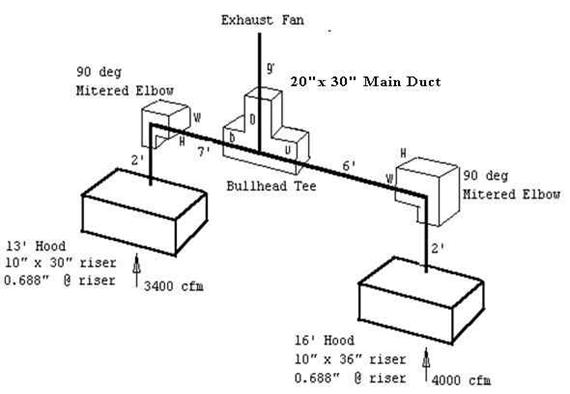The first step is to break the system into sections.

Section 1 runs from the 16’ Hood to the Bullhead Tee.

Section 2 runs from the 13’ Hood to the Bullhead Tee.

Section 3 runs from the Bullhead Tee to the Exhaust Fan.

Now calculate the pressure losses for each section.

Section 1

Air Flow Rate  Q = 4000 cfm

Cross-Sectional Area  A = 10 x 36/144 = 2.5 ft2

Center Line Distance  L = 2’ + 6’ = 8’

Velocity  V = 4000/2.5 = 1600 ft/min

Velocity Pressure = Pv1 = (V/4005)2  = (1600/4005) 2 = 0.16”

Loss Calculations

Component Losses

Hood Loss

Phood1 =  -0.688”

Look up from manufacturer hood static pressure curves.  Here is a link to the Hood Static Pressure Calculator.

Frictional Losses

Use the Friction Chart to look up the pressure loss per 100 ft of duct.

Pfr1 = -(.16”/100 ft) * (8’) = -0.013”

Dynamic Losses

Mitered Elbow.  Look up Fitting 3-6 in Appendix 2 - ASHRAE Fittings.  The dynamic coefficient C0 = 1.3

Pelbow1  = - Pv1 = -(1.3)*(0.16”) = -0.208”

Bullhead Tee.  Look up coefficient from Appendix 3 - Bullhead Tee Curves.

Some general rules for bullhead tees: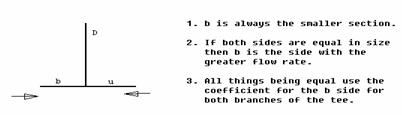Since Section 1 has a larger duct size, this section is the “u side of the bullhead tee.  The following describes how to use the bullhead tee curves to find Ku for the “u side of the bullhead tee.

Since AU /AD = (10x36)/(20x30) = .6, we find the bullhead tee curves for which AU /AD is .6 and the y-axis represents KU.
We know that Qb /QD = 4000/(4000+3400) = .54.  For simplicity and ease of graphing, we round .54 to the nearest 10th giving us .5.
We also know that Ab /AD = (10x30)/(20x30) = .5.
Equipped with these ratios, can draw a line from the point on the x-axis where Qb /QD is .5  up to where it intersects the curve for which Ab /AD is .5
We find Ku = 1.6
NOTE: Due to human error resulting from manually graphing the value of KU , the number you graph may be slightly different than the value show above.  The important thing is to know how to use the curves and get a reasonable value for KU.

Now we can calculate the pressure drop contributed by the bullhead tee for Section 1:

Pbulltee1 = -Ku * Pv1 = -(1.6)*(0.16”) = -0.256”

The total pressure loss for Section 1 is:

P t loss 1 = Phood1  + Pfr1 + Pelbow1 + Pbulltee1

P t loss 1 = -0.688” -0.013” -0.208” -0.256” = -1.165”

Section 2

Air Flow Rate  Q = 3400 cfm

Cross-Sectional Area  A = 10 x 30/144 = 2.1 ft2

Center Line Distance  L = 2’ + 7’ = 9’

Velocity  V = 3400/2.1 = 1619 ft/min

Velocity Pressure = Pv2 = (V/4005)2  = (1619/4005) 2 = 0.16”

Loss Calculations

Component Losses

Hood Loss

Phood2 =  -0.688”                   Look up from hood static pressure curves.

Frictional Losses

Use the Friction Chart to look up the pressure loss per 100 ft of duct.

Pfr2 = -(.18”/100 ft) * (9’) = -0.016”

Dynamic Losses

Mitered Elbow.  Look up Fitting 3-6 in Appendix 2 - ASHRAE Fittings.

The dynamic coefficient C0 = 1.3

Pelbow2  = - Pv2 = -(1.3)*(0.16”) = -0.208”

Bullhead Tee.  Using the methodology described for the bullhead tee in Section 1, we can find the value of the coefficient, Kb, for the “b side of the bullhead tee.  Use the bullhead tee curves for which AU /AD is .6 and the y-axis represents Kb.

We find that Kb = 1.75 and the resulting pressure loss is:

Pbulltee 2 = -Kb * Pv2 = -(1.75)*(0.16”) = -0.280”

The total pressure loss for Section 2 is:

P t loss 2 = Phood2  + Pfr2 + Pelbow2 + Pbulltee2

P t loss 2 = -0.688” -0.016” -0.208” -0.280” = -1.192”

Balance by Design

Note that the pressure loss of Section 2 is greater than the loss of Section 1.  To balance the system by design increase the air flow rate in Section 1 to bring it up to the higher pressure loss of Section 2.

To correct the air flow rate for Section 1 use the Fan Laws:

Q 1 new = Q 1 old * (P t loss 1 new/ P t loss 1 old)1/2

Q 1 new = 4000 * (1.192/1.165)1/2 = 4046 cfm

Section 3

Air Flow Rate  Q = 3400 cfm + 4046 cfm = 7446 cfm

Cross-Sectional Area  A = 20 x 30/144 = 4.17 ft2

Center Line Distance  L = 9’

Velocity  V = 7446/4.17 = 1785 ft/min

Velocity Pressure = Pv3 = (V/4005)2  = (1785/4005) 2 = .20”

Loss Calculations

Component Losses

None

Frictional Losses

Use the Friction Chart to look up the pressure loss per 100 ft of duct.

Pfr2 = -(.15”/100 ft) * (9’) = -0.014”

Dynamic Losses

None

Total pressure loss for Section 3 is:

P t loss 3 =  Pfr3

P t loss 3 =  -0.014”

Total Pressure Loss of System

Since the pressure loss of Section 2 is greater than that of Section 1, it is used to calculate the pressure loss of the entire system as shown below:

P t loss = P t loss 2 + P t loss 3  = -1.192” -0.014” = -1.206”

Now we will change the air flow rate through Section 2 from 3400 CFM to 3000 CFM.  We will illustrate how once you know one (CFM, S.P.) point of a system you can use the Fan Laws to calculate the pressure loss for other air flow rates.

Section 1

There is no change.    P t loss 1  = -1.165”

Section 2

Air Flow Rate  Q = 3000 CFM

Cross-Sectional Area  A = 10 x 30/144 = 2.1 ft2

Center Line Distance  L = 2’ + 7’ = 9’

Velocity  V = 3000/2.1 = 1429 ft/min

Velocity Pressure = Pv2 = (V/4005)2  = (1429/4005) 2 = 0.13”

Loss Calculations

Component Losses

Hood Loss.   Use the Fan Laws to calculate a new Hood Loss or look it up in the Hood S.P. chart.

Phood2 = -(0.688”)*((3000 CFM)2/(3400 CFM)2)

Phood2 =  -0.536”

Frictional Losses

Use the Friction Chart to look up the pressure loss per 100 ft of duct.

Pfr2 = -(.15”/100 ft) * (9’) = -0.014”

Dynamic Losses

Mitered Elbow.  Look up Fitting 3-6 in Appendix 2 - ASHRAE Fittings.

The dynamic coefficient C0 = 1.3

Pelbow2  = - Pv2 = -(1.3)*(0.13”) = -0.169”

Bullhead Tee.  Since Section 2 is the “b” side, we use the set of bullhead tee curves for which AU /AD is .6 and the y-axis represents Kb.

We find that Kb = 1.65

Pbulltee 2 = -Kb * Pv2 = -(1.65)*(0.13”) = -0.215”

Total Section Loss:

P t loss 2 = Phood2  + Pfr2 + Pelbow2 + Pbulltee2

P t loss 2 = -0.536” -0.014” -0.169” -0.215” = -0.93”

Using the Fan Laws to calculate the new total pressure loss for Section 2:

P t loss 2 = -(1.192”)*((3000 CFM)2/(3400 CFM)2) = -0.93”

Balance by Design

Note that the pressure loss of Section 1 is now greater than the loss of Section 2.  To balance the system by design we must increase the air flow rate in Section 2 to bring it up to the higher pressure loss of Section 1.

To correct the air flow rate for Section 2 use the Fan Laws:

Q 2 new = Q 2 old * (P t loss 2 new/ P t loss 2 old)1/2

Q 2 new = 3000 * (1.165/0.93)1/2 =  3357 CFM

Section 3

Air Flow Rate  Q = 3357 CFM + 4000 CFM = 7357 CFM

Cross-Sectional Area  A = 20 x 30/144 = 4.17 ft2

Center Line Distance  L = 9’

Velocity  V = 7357/4.17 = 1764 ft/min

Velocity Pressure = Pv3 = (V/4005)2  = (1764/4005) 2 = 0.19”

Loss Calculations

Component Losses

None

Frictional Losses

Use the Friction Chart to look up the pressure loss per 100 ft of duct.

Pfr2 = -(0.14”/100 ft) * (9’) = -0.013”

Dynamic Losses

None

Using the Fan Laws to calculate the new total pressure loss for Section 3:

P t loss 3 = -(0.014”)*((7357 cfm)2/(7446 cfm)2) = -0.013”

Total System Loss

Calculated with Tables and ASHRAE Charts

P t loss = P t loss 1 + P t loss 3  = -1.165” -0.013” = -1.178”

As shown above, Branch 1 of the junction is used to calculate the system’s total pressure loss because it has the greater pressure drop of the two branches.

Calculated with the Fan Laws

P t loss = -(1.206”)*((7357 cfm)2/(7446 cfm)2) = -1.178”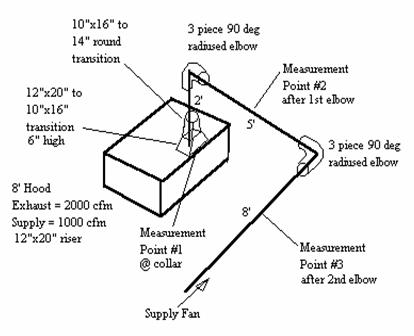The first part of the problem will show the pressure gains obtained from measuring the total pressure at 3 points shown in the diagram above.  It will provide some rules of thumb for estimating pressure for elbow and at the supply collar.  The second part of the problem will calculate the pressure gain of the system and compare it to the measured pressure gain.

The entire system satisfies the definition of a section since there are no junctions or duct size changes.  The transitions off the supply collars can be included in the section.

Supply System - Measured Pressure

A 0” to 1” Dwyer manometer was used to measure the pressure of the system at 3 points.  The pressure was measured for two different flow rates.  The results are show in the table below.

Measurements Taken at 3 points of the Supply System

 Air Flow Rate (CFM) Velocity (ft/min) Point 1 @ collar (in. wg) Point 2 after 1st elbow (in. wg) Point 3 after 2nd elbow (in. wg) 1000 935 0.075 0.140 0.260 1920 1793 0.276 0.570 0.910

The table shows:

1)          How high air velocities greatly increase the pressure.  When the air flow rate is raised to 1920 cfm, the velocity through the duct about doubles and the pressure increases 3-1/2 fold.

2)          The system effect of having 2 elbows close to each other and being close to the hood.
Using the pressure gains for 1000 cfm flowing through the system, we see that the pressure gain for the first elbow is: 0.14” - 0.075” = 0.065”.  This reflects the system effect of having an elbow close to the supply opening of a hood.
The pressure gain for the second elbow is:  0.26” - 0.14” = 0.12”.  This reflects the system effect of having two elbows within close proximity to one another and being close to the hood.

3)          When the system supplies 1000 CFM, the pressure gain at the supply collar is 0.075”.  This illustrates how low the pressure really is when a system is designed for the desired velocity between 900 and 1000 ft/min.  The table below provides some rules of thumb when estimating pressure gain at the supply collar:

 Hood Length (L) Pressure Loss Estimate L <= 8’ 1/16” max. 8’ < L <= 12’ 1/16” to 1/8” max. 12 < l <= 16’ 1/8” to 1/4” max.

This table assumes that the system has been designed for velocities around 1000 ft/min.

Test Kitchen Supply System - Calculated Pressure

Section 1

Air Flow Rate  Q = 1000 cfm

Cross-Sectional Area  A = pr2 = p (7)2/144 = 1.069 ft2

Center Line Distance  L = 15’

Velocity  V = 1000/1.069= 935 ft/min

Velocity Pressure = Pv1 = (V/4005)2  = (935/4005) 2 = 0.055”

Loss Calculations

Component Losses

Hood.  Assume 1/16” pressure gain at the collar.

P hood = 0.063”

Frictional Losses

Use the Friction Chart to look up the pressure loss per 100 ft of duct.

Pfr = -(0.095”/100 ft) * (15’) = 0.014”

Dynamic Losses

12”x 20” to 10” x 16” Rectangular Transition

To find the dynamic coefficient we calculate:

q/2 = tan -1(2”/6”) = 18o   Therefore: q = 36 o

A0 / A1 = (12x20)/(10x16) = 1.5

Therefore C0 = 0.05

P trans1 = C0Pv1 = (0.05)(0.055”) = 0.003”

10”x 16” Rectangular to 14” Round Transition

B = W/H(A0 / A1 )2 = (16/10)(1.069/1.111)2 = 1.48

Re = 8.56DV = (8.56)(14”)(935 ft/min) = 11205

Therefore Re x 10-4 = 11.  Use the value for Re x 10-4 = 10

L/D is not relevant in this case.

C0 = 0.11

P trans2 = C0Pv1 = (0.11)(0.055”) = 0.006”

Use ASHRAE fitting 3-2.  Assume a 3 piece elbow.

Assume r = 10”. So, r/D = 10/14 = .71 therefore Kq = 1.

C0 = 0.54

P elbow1 = C0Pv1 = (0.54)(0.055”) = 0.03”

P elbow2 = C0Pv1 = (0.54)(0.055”) = 0.03”

We must figure in the system effect incurred by having an elbow close to the supply collar.  Use the table in the System Effect section of this paper to estimate the system effect.

The elbow is about 2’ above the supply riser to KSE = 1.75.

P SE elbow-hood = KSE P elbow1 = (1.75)(0.03”) = 0.053”

Now we must factor in the system effect for the 2 elbows in succession.  We decide to the S-Shaped fitting in the ASHRAE handbook to estimate the system effect.  We use ASHRAE fitting 3-14.

q = 90 o

L/D = 60”/14” = 4.29

K SE = 1.55

P SE S-fitting = K SE(P elbow1 + P elbow2) = 1.55(0.03” + 0.03”) = 0.093”

Total Section Loss:

P t loss 1 = Phood  + Pfr + Ptrans1 + Ptrans2  + P SE elbow-hood + P SE S-fitting

P t loss 1 = 0.063” + 0.014” + 0.003” + 0.006” + 0.053” + 0.093”

P t loss 1 = 0.232”

The measured value of 0.26” differs because of error in the system effect estimates.

Now we can determine the size fan we need.  A 10” blower will handle 1000 cfm at 0.232”.

To calculate the Fan Static Pressure:

P s fan = P t loss - P v discharge

Use the blower manufacturer product literature to get the dimensions for the blower outlet so the velocity pressure at the fan discharge can be calculated:

P v discharge = (V discharge/4005)2

P v discharge = ((1000/((11.38*13.13)/144))/4005)2

P v discharge = 0.058”

P s fan = 0.232” - 0.058” = 0.174”

Total Pressure (P T)

P T = P v + P s
P
v = Velocity Pressure
P
s = Static Pressure

Fan Static Pressure (P s fan)

For Exhaust:
P
s fan = P t loss

For Supply:
P
s fan = P t loss - P v discharge

P s fan = Fan Static Pressure

P t loss = Dynamic and Friction Losses

P v discharge = Velocity Pressure at the Fan Discharge

Velocity Pressure (P v)

P v = r(V/1097)2
For standard air P v equals:
P
v = (V/4005)2
V = Velocity through the duct.

Friction Losses (P fr)

Darcy-Weisbach Equation
P
fr = (f / D) x L x VP

Then substitute (f / D) with H f:

P fr = Hf x L x VP
L = Duct Section Length (ft)
f = Friction Factor
D = Duct Diameter (ft)

H f  is defined as:
H
f =  aVb / Qc
V = Velocity through the duct cross section.

Q = Flow Rate (cfm) through the duct section.

See Table titled Surface Roughness Correlation Constants to get values for a,b, and c.

Surface Roughness Correlation Constants

 Material k A b c Aluminum, Black Iron, Stainless Steel 0.00015 0.0425 0.0465 0.602 Galvanized 0.0005 0.0307 0.533 0.612 Flexible Duct 0.003 0.0311 0.604 0.639

k = Roughness factor for the material.

Fitting 3-2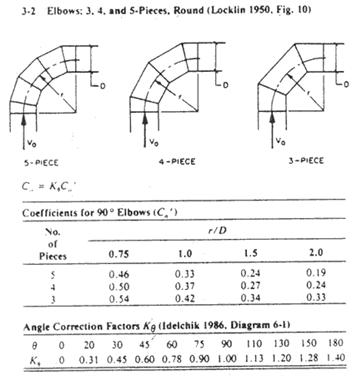Fitting 3-6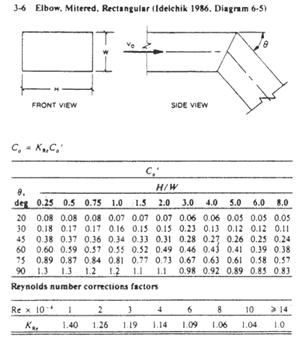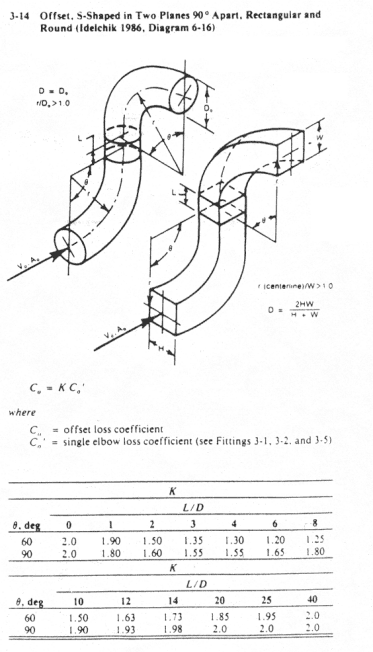Fitting 4-6